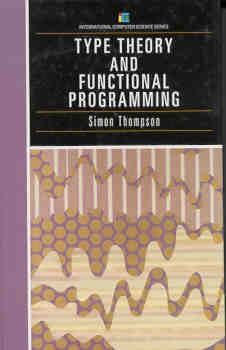## Simon Thompson

### Errata

Chapter 1, Exercise 1.4 part 1, should ask for a proof of

(A => B) => (not B => not A)


Chapter 1, Exercise 1.5, should ask for a proof of

(A \/ B) => ((A => _|_)/\(B => _|_) => _|_)


Page 39, Theorem 2.14: Delete "indexNormalisation theorem!untyped \lambda- calculus": it's a mistake in the underlying LaTeX code.

Page 46, Def 2.23: The first sentence ends with "if", and the following bullet points each begins with "If": remove these latter "If"s.

Page 48, Def 2.26: "A s-instance ..." should be "An s-instance ...".

Page 99, exercise 4.19: The references to 0_n should be to 1_n.

Page 102, paragraph 3: "recommeding" should be "recommending"

Page 103: The order of arguments in the definitions of add and mult are wrong: they should be (respectively): λm.λn. prim n m (λp.λq. (succ n)), and λm.λn. prim n 0 (λp.λq. (add m q)).

Page 104: The two argument Ackermann function is incorrect. The second case should be ack (m+1) 0 = ack m 1, not ack (m+1) 0 = 1.

Similarly, in the definition of iter on the same page, the base case should be iter f 0 = f 1, not iter f 0 = 1. Finally, the term of iter should then be: λ f(N→N).λ nN.(prim n (f 1) (λ p.λ q.(f q))).
Note, the final ack term misses a pair of parenthesis: λ n.(prim n succ λ p.λ g.(iter g)) ought to be λ n.(prim n succ (λ p.λ g.(iter g))).

Page 107: In the middle of the page it says "The preceding type is enclosed in inverted commas as it is not a Miranda type" but the inverted commas actually are missing.

Page 117, section 4.10.4 This section isn't correct: there's no way of deriving the final judgement here from the rules.

Page 136, exercise 5.9: This should read "Give definitions of addition and subtraction over the integers, and prove ..."

Page 152, paragraph beginning "Now": Insert "namely" before "the supercombinator abstraction".

Page 152, last paragraph: In "There is a single m f e in the ...", the "m f e" should be non-emphasised and be without inner spaces.

Page 166, last paragraph: Missing ellipsis after d : (\forall x_1:A_1)..

Page 190, paragraph 3: The reference to Gödel 58 looks quite odd with umlauts over the 5. LaTeX artefact.

Page 201, exercise 6.4: The exercise asks for a proof showing that lt_1 m n and lt_2 m n are equivalent proposition. It should instead ask for a proof showing I(bool, lt_1 m n, True) and lt_2 m n as equivalent.

Page 210, last paragraph: "oredering" should be "ordering".

Section 6.2: There's a problem here in the correctness of the algorithm! Specifically it comes from the treatment of lesseq and greater. Since lesseq x y means that x is less than or equal to y, the effect of the algorithm is to sort the list in descending order, because filter (lesseq x) filters out the elements which are greater than or equal to x!. The culprit among the lemmas in Lemma 6.2 is (5) (and the corresponding result for greater).

Page 213, line -2: Incomplete sentence: should read "... predecessor of (f x) in B."

Page 217, first sentence in sec 6.3.3: Missing space, "... quantifier \foralldefines ..." should be "... quantifier \forall defines ...".

Page 250, 264, and 267: Goteborg should be Göteborg (Swedish name) or Gothenburg (English name).

Page 267, middle of page: says "{ x : A | P (x) } prop", but should say instead that "{ x : A | P (x) } is a type".

Page 274, paragraph 3: The second "the" in "... we behave in the same way as for the an element of type A ..." should be removed.

Page 300, paragraph 1: "... we have give a way ..." should be "... we have given a way ..."

Page 316, Definition 8.1: "A full definition the system ..." should be "A full definition of the system ..."

Page 336, paragraph 2: "Indeed, it can shown consistent ..." should be "Indeed, it can be shown consistent ..."

Thanks for Francesco Mazzoli, Robert Helgesson, Iosif Neitzke, Marvin D Hernandez, Gavin Mendel-Gleason, Michele Morelli and Eugene Akentyev for pointing out these problems.